Courses

# Match the following Question of Definite Integrals and Applications, Past year Questions JEE Advance JEE Notes | EduRev

## JEE : Match the following Question of Definite Integrals and Applications, Past year Questions JEE Advance JEE Notes | EduRev

The document Match the following Question of Definite Integrals and Applications, Past year Questions JEE Advance JEE Notes | EduRev is a part of the JEE Course Maths 35 Years JEE Mains & Advance Past year Papers Class 12.
All you need of JEE at this link: JEE

Match the Following

DIRECTIONS (Q. 1 and 2) : Each question contains statements given in two columns, which have to be matched. The statements in Column-I are labelled A, B, C and D, while the statements in ColumnII are labelled p, q, r, s and t. Any given statement in Column-I can have correct matching with ONE OR MORE statement(s) in Column-II. The appropriate bubbles corresponding to the answers to these questions have to be darkened as illustrated in the following example :

If the correct matches are A-p, s and t; B-q and r; C-p and q; and D-s then the correct darkening of bubbles will look like the given.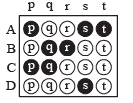Q. 1. Column I                                                                      Column II

(A)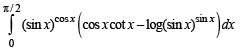(p) 1

(B) Area bounded by –4y2 = x and x – 1 = –5y2                  (q) 0

(C) Cosine of the angle of intersection of curves y = 3x – 1
log x and y = xx – 1is                                                           (r) 6 ln 2

(D) Let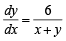where y(0) = 0 then value of y when
x + y = 6 is                                                                             (s) 4/3

Ans. (A) - p ; (B) -s ; (C) - p ; (D) - r

Solution.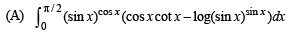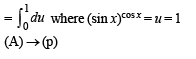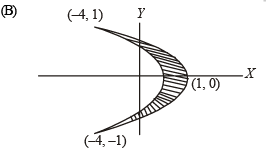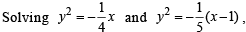we get intersection points as (– 4, + 1)

∴ Required area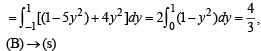(C) By inspection, the point of intersection of two curves y = 3x–1 log x and y = xx – 1 is (1, 0)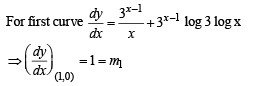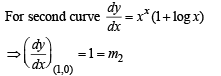∵ m1 = m2 ⇒ Two curves touch each other

⇒ Angle between them is 0°

∴ cos θ = 1,

(C) ®→ (p)

(D)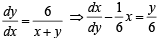I.F. = e–y/6

⇒ Solution is xe–y/6 = – ye–y/6 – 6e–y/6 + c
⇒ x + y + 6 = cey/6
⇒ x + y + 6 = 6ey/6 ∴  (y (0) = 0)
⇒ 12 = 6ey/6 (using x + y = 6)
⇒ y =  6 ln 2 (D) → (r)

Q. 2. Match the integrals in Column I with the values in Column II and indicate your answer by darkening the appropriate bubbles in the 4 × 4 matrix given in the ORS.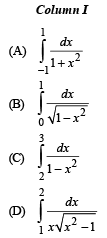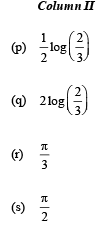Ans. (A) - s ; (B) -s ; (C) - p ; (D) - r

Solution.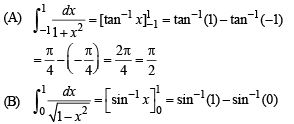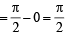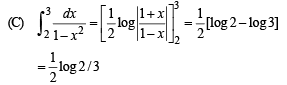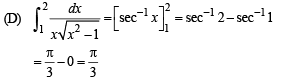Q. 3. DIRECTIONS (Q. 3) : Following question has matching lists. The codes for the list have choices (a), (b), (c) and (d) out of which ONLY ONE is correct.

List - I                                                                                                  List - II

P. The number of polynomials f (x)                                                1. 8
with non-negative integer coefficients
of degree < 2, satisfying f (0) = 0 and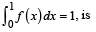Q. The number of points in the interval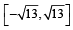2. 2
at which f (x) = sin(x2) + cos(x2) attains its maximum
value, is

R.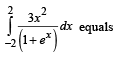3. 4

S.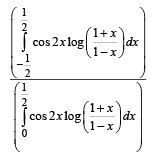4. 0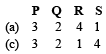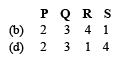Ans. (d)

Solution. P(2) Let f (x) = ax2 + bx + c
where a, b, c > 0  and a, b, c are integers.
∵ f (0) = 0 ⇒ c = 0
∴f (x) = ax2 + bx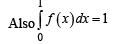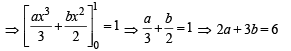Q∵ a and b are integers
a = 0 and b = 2
or a = 3 and b = 0

∴ There are only 2 solutions.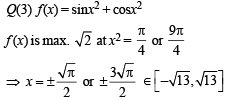∴ There are four points.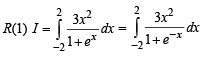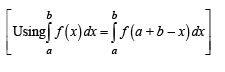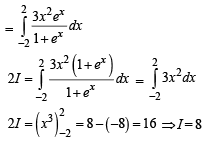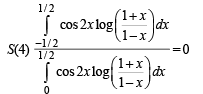∵ Numerator = 0, function being odd.

Hence option (d) is correct sequence.

Offer running on EduRev: Apply code STAYHOME200 to get INR 200 off on our premium plan EduRev Infinity!

63 docs|43 tests

,

,

,

,

,

,

,

,

,

,

,

,

,

,

,

,

,

,

,

,

,

,

,

,

;